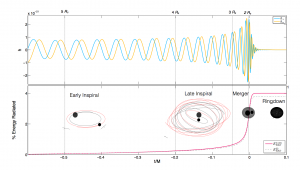# BINARY BLACK HOLE SIMULATIONS

Welcome to the Georgia Tech catalog of binary black hole simulations. The publication describing this catalog can be found on Classical & Quantum Gravity “Georgia tech catalog of gravitational waveforms”

The catalog contains 452 unique simulations. These simulations can be used to analyze the dynamics and data-analysis for gravitational-waves from a binary black hole coalescence. In this site you will find a information about:

1. Table summarizing the binary black hole parameter space of the catalog
2. Gravitational-waveform for a given simulation and relevant scripts
3. Raw simulation data
4. Technical details about the simulations————————-
CATALOG TABLE

The table summarizing the catalog of our simulations (initial parameters of binary black holes and remnant black hole) can be downloaded here

LINK TO INTERACTIVE CATALOG TABLE (with waveform download option)

————————-
GRAVITATIONAL WAVEFORMS

To download ready to use gravitational-waveforms for entire catalog click here

These waveform are in LSC-hdf5 format and can be scaled to a given total-mass and distance. The scripts to read these waveforms can be found here.

————————-
TECHNICAL INFORMATION

• In each simulation, binary black holes are scaled to total mass set by the apparent horizon mass at the start of the simulation (ideally, Mtot = 1).
• All gravitational-waveforms (LSC-hdf5 format) contain radiated modes l=2:6, m=-l:l. The waveforms are computed at finite extraction radius and finite resolution. Initial junk radiation is removed from these waveforms.
• Waveforms from multiple extraction radius and individual modes are available in raw simulation data.
• Spins, masses and momenta are defined at the start of the simulation.

————————-
Key Words

GTID := Permanent ID for each simulations
q := mass-ratio of black holes (m1/m2 >=1)
(a1x, a1y, a1z) := dimensionless spin on bigger black hole
(a2x, a2y, a2z) := dimensionless spin on smaller black hole
GT-tag := Internal directory name